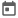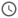##### 18 Sep 2021##### 4 mins

When we write a code, we may need to use some line of code repeatedly in various places. Writing the same line of code leads to errors in code and decreases the readability of the code. Thus to execute the same code several times, there are functions in a programming language (also known as a subroutine). In Verilog, there are two types of subroutines, functions and tasks. A function is the same as functions in any programming language, but tasks are slightly different. Let us see them in detail.

### What are subroutines?

Before learning more about functions and tasks, let us see what a subroutine is. A routine defines the execution flow of a code. A subroutine is some code that is executed only when called by the main programme. Once the execution of the subroutine is complete, the pointer moves back to the main programme.

### Functions

A function, as discussed earlier, is the same as that found in other programming languages. To write a function, first, we need to declare it. This step is known as a functional declaration. After declaration, we write the function's body, which is nothing but the function's functionality and is known as a function definition. Please remember, we cannot define a function before declaring it.

Function declaration consists of 3 parts:

1. Function name – This is the function's name that will be used to call the function. The name can be any valid identifier.
2. Automatic - This keyword is used to make the function automatic. Detailed explanation about automatic function is discussed later in this lesson.
3. Return type – This is the data type of the value that is returned by the function. If no value needs to be returned, then void is used.
4. Arguments – This is the input to the functions. There can be no or even more than one argument in a function. Arguments are similar to the port list in modules.

A function is declared using a `function` keyword, and the function definition ends with the `endfunction` keyword. The function definition goes inside `begin end` block.

#### Syntax

``````function [automatic] [return_type] name ([arguments]); // Function Declaration
begin
// function definition goes here
end
endfunction``````

#### Example

``````module function_demo;
reg b;
function reg print(input reg [3:0] data);
begin
\$display("data is %0b", data);
print = 1;
end
endfunction

// This function declaration is not valid as
// there is no argument.
/**
function reg print1();
begin
\$display("data is");
print1 = 1;
end
endfunction **/

initial begin
/** `if` is used here because function will return a value
Thus, the returned value needs to be assigned or used in
conditional statement. If it is not assigned then Verilog
will treat it as a task and throw an error saying task print
is not declared **/
if(print(4'b1100)) begin
\$display("printed successfully");
end
// This is also valid as returned value is assigned to variable
b = print(4'b1010);

// This is not valid
// print(4'b1010);
end
endmodule``````
##### Output
``````# data is 1100
# printed successfully
# data is 1010``````

#### Rules to remember

In Verilog, while declaring a function, some rules need to be remembered. These rules are:

1. A function should have at least one argument.
2. Function argument can only have `input` as direction. `output` or `inout` cannot be used in function arguments.
3. Function definition should have any delay statement or `@` statements, as functions cannot consume time.
4. Function definition should not have a non-blocking assignment or procedural-continuous assignment.

In Verilog, a task is a particular subroutine type, which can consume time. As we know, Verilog is a time-dependent simulator. Thus, in some cases, we need to provide a delay in subroutines. However, delays cannot be used in functions as they should not consume time; otherwise, a compile-time error is shown. A task helps to tackle this problem.
Similar to functions, a task is also declared first and then defined. However, a task declaration does not consist of a return type. Instead, we use a port with output direction in the arguments.

In Verilog, global functions or tasks are invalid. It is only valid in System Verilog. Declaring a global function or task will give a compile-time error. The concept of global declaration will be discussed in System Verilog. This fact is mentioned here as people tend to confuse whether they can use global declaration in Verilog.

#### Syntax

``````task [automatic] name ([arguments]);
begin
end

#### Example

``````module task_demo;
reg [3:0] op;
begin
\$display("At [%0t] printing from task", \$time);
\$display("At [%0t], output from task = %b", \$time, op);
end

// Task with 2 ip arguments and 1 output argument. As task calculates
// sum thus it needs to return a value. Function cannot
// be used as we want to give some delay also.
task sum(input reg[3:0] a, b, output reg [3:0] sum);
begin
\$display("At [%0t], Calculating sum...", \$time);
#10;
sum = a + b;
end

initial begin
// As there is no return type, thus tasks are not
// assigned to any variable. Rather, the variable in which
// output needs to be assigned is passed as an argument.
sum(4'b1010, 4'b0101, op);
print();
end
endmodule``````
##### Output
``````# At , Calculating sum...
# At  printing from task
# At , output from task = 1111``````